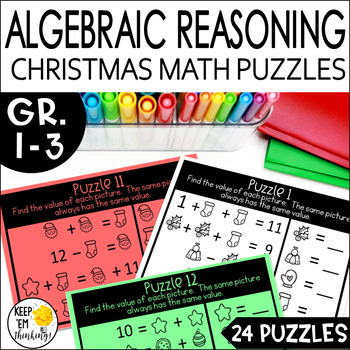digital

# Christmas Math Logic Puzzles | Algebraic Reasoning | DIGITAL AND PRINTSubject
Resource Type
Format
Zip (3 MB|42 pages)
Standards
\$4.00
\$4.00The Teacher-Author indicated this resource includes assets from Google Workspace (eg. docs, slides, etc.).

#### Also included in

1. This is a bundle of my five CHRISTMAS CRITICAL THINKING PRODUCTS for grades 1-3. This bundle teaches logic and deductive reasoning, analogies and classifying and attribute listing to get your kiddos thinking at those higher levels. This bundle is completely updated with new clip art and fonts!Purch
\$14.40
\$18.00
Save \$3.60

### Description

UPDATED! I just added a digital version of the puzzles. Want some fun Christmas math activities for 1st - 3rd grade? These Christmas Addition and Subtraction Math Logic Puzzles are perfect for early finishers or students who have already mastered their basic addition and subtraction facts. This set of 24 addition and subtraction logic puzzle task cards helps your students become effective problem solvers.

Each task card contains 4 problems using pictures and numbers. The students must figure out the value of each picture. Students are using addition and subtraction within 20 to solve problems with unknowns in all positions. They are working with equations with a symbol for the unknown number to represent the problem.

These task cards are a fun way to challenge your high achievers and are perfect in a center or for early finishers. These activities lay the foundation for problems in which students use variables in mathematical expressions.

All the task cards come two to a page and are in black and white to save you ink! They look great printed on Astrobrites green and red paper. There are answer keys and recording sheets included.

MY FAVORITE WAYS TO USE THESE TASK CARDS:

• Morning Warm-up Activities
• In a center
• Think - Pair - Share
• For early finishers

These task cards align with the Operations and Algebraic Thinking domain in the Common Core. They specifically address the following standards:

• 1.OA.A.1 and 1.OA.A2 Represent and solve problems involving addition and subtraction
• 1.OA.B.3 and 1.OA.B.4 Understand and apply properties of operations and the relationship between addition and subtraction
• 1.OA.C.5 1.OA.C.6 Add and subtract within 20
• 1.OA.D.7, 1OA.D.8 Add and subtract within 20
• 2.OA.A.1 Represent and solve problems involving addition and subtraction
• 2.OA.B.2 Add and subtract within 20

OTHER PRODUCTS YOU MIGHT BE INTERESTED IN:

Want to save \$\$\$ Bundle Up!

This resource is also included in my Christmas Critical Thinking Bundle.

Follow me to be notified when I post a new product. All my products are 50% off for the first 48 hours, so it pays to be a follower!

TERMS:

Copyright © Keep ‘em Thinking!, Susan Morrow. This product is intended for classroom and personal use ONLY and may be used only by the original downloader Copying for more than one teacher, classroom, department, school, or school system is prohibited. You may not distribute or display this product digitally for public view. Failure to comply is a copyright infringement and a violation of the Digital Millennium Copyright Act (DMCA).

Remember to Always ... Keep 'em Thinking!
Susan Morrow

Connect with Me

Total Pages
42 pages
Included
Teaching Duration
N/A
Report this Resource to TpT
Reported resources will be reviewed by our team. Report this resource to let us know if this resource violates TpT’s content guidelines.

### Standards

to see state-specific standards (only available in the US).
Fluently add and subtract within 20 using mental strategies. By end of Grade 2, know from memory all sums of two one-digit numbers.
Use addition and subtraction within 100 to solve one- and two-step word problems involving situations of adding to, taking from, putting together, taking apart, and comparing, with unknowns in all positions, e.g., by using drawings and equations with a symbol for the unknown number to represent the problem.
Determine the unknown whole number in an addition or subtraction equation relating three whole numbers. For example, determine the unknown number that makes the equation true in each of the equations 8 + ? = 11, 5 = ▯ - 3, 6 + 6 = ▯.
Understand the meaning of the equal sign, and determine if equations involving addition and subtraction are true or false. For example, which of the following equations are true and which are false? 6 = 6, 7 = 8 - 1, 5 + 2 = 2 + 5, 4 + 1 = 5 + 2.
Add and subtract within 20, demonstrating fluency for addition and subtraction within 10. Use strategies such as counting on; making ten (e.g., 8 + 6 = 8 + 2 + 4 = 10 + 4 = 14); decomposing a number leading to a ten (e.g., 13 - 4 = 13 - 3 - 1 = 10 - 1 = 9); using the relationship between addition and subtraction (e.g., knowing that 8 + 4 = 12, one knows 12 - 8 = 4); and creating equivalent but easier or known sums (e.g., adding 6 + 7 by creating the known equivalent 6 + 6 + 1 = 12 + 1 = 13).